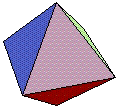## STAT 10

(Sec. 5a-5c)

Introduction to Statistical Reasoning

## Instructor: Ivo Dinov, Asst. Prof.

Departments of Statistics & Neurology

Due Date:

# Friday, Mar. 08, 2002, turn in after lecture

See the HW submission rules . On the front page include the following header .

• (HW_6_1)  Let X ~ Binomial(10, 0.3). Write down the 3 requirements for using a Binomial distribution model. Under what conditions can we relax these requirements and use Binomial approximation ? Write the formula for the binomial probabilities and define every term you use. Compute the probability of the event A={1<= X<4}. Let B be the event B={4< X<=10}. Which is bigger, P(A) or P( B)? [Hint: You are free to use the Statistical Online Compute Resource ( SOCR) that we have available from the class web-page to calculate these probabilities. However, you still need to write down the actual formulas for the Binomial probabilities - see our class notes.]

• (HW_6_2) Six fair, but unusual, octahedral (8 face) dice are rolled twice. Let Y be the random variable representing the total sum from this experiment (each die turns up an integer value between 1 and 8). Find the expected value of Y, mY, and the standard deviation of Y , sYSuppose, we carry this experiment (rolling six dice twice) 11 times. What would be approximately the distribution of the sample average, Y ¯? What are the mean and the standard deviation of the sample average? [Note: Not all types of dice, any number of faces, are possible to construct. For a nice description of which ones are possible see the following web-page .]

• (HW_6_3) One of the oldest mnemonic techniques is known as the method of loci. Past experience suggests this method could be a useful technique for students who want to be able to remember an ordered set of points for an essay type question in an exam. The first step in this technique is to think of a set of locations that are naturally ordered. For example the route you commonly use to get from home to UCLA. Along this route are various familiar locations such as the bus stop where you catch the bus. You will require as many familiar locations as the number of points you wish to remember. The next step is to associate (in order) each location with one item you wish to remember. When you need to recall the points simply travel (in your mind) along the route, retrieving each item as you reach its location.
An experiment with two methods of instruction (normal and mnemonic) was conducted to evaluate the mnemonic method. Thirty-eight participants were randomly assigned to one of the two methods. Eighteen were given 24 words and instructed simply to "try and remember" each so that they could later recall the words. ( Normal controls). The second group of 20 participants studied the same 24 words but were instructed in the method of loci (Mnemonic group). One day later, participants were tested by being asked to recall the list of 24 words. The response measured was the number of words correctly recalled out of 24. The results are as follows:

 Controls: 9 7 5 9 7 5 11 7 9 10 15 13 13 8 7 12 12 7 Mnemonic group: 11 14 14 16 12 16 9 13 14 15 17 15 14 20 14 15 14 16 13 10

• What are the mean and standard deviation for the group of participants who received mnemonic instructions? How about the mean and standard deviation for the group of participants who received normal instructions?

•
• Find a 95% confidence interval for the difference in the mean number of words recalled between the normal and mnemonic instruction methods. Interpret your result using plain English in a couple of sentences.

•
• What sample-size would yield a CI of half the size you found above?

•
• Does your confidence interval above contain the true difference? Explain your answer briefly using plain English in 2-3 sentences.

\Ivo D. Dinov, Ph.D., Departments of Statistics and Neurology, UCLA School of Medicine/